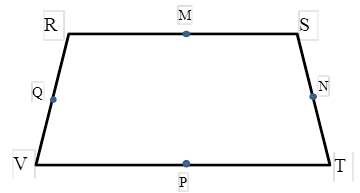Chapter 4.4, Problem 10EElementary Geometry For College St...

7th Edition
Alexander + 2 others
ISBN: 9781337614085

Solutions

Chapter
SectionElementary Geometry For College St...

7th Edition
Alexander + 2 others
ISBN: 9781337614085
Textbook Problem

For Exercises 9 and 10, consider isosceles trapezoid RSTV with R S ¯ | | V T ¯ , and midpoints M, N, P, Q of the sides.Does a) Q N = 1 2 ( R S + V T ) ? .b) M P = 1 2 ( R V + S T ) ? .Exercises 9, 10

To determine

a)

To Explain:

Does QN=12(RS+VT)?

Explanation

Consider the following isosceles trapezoid RSTV.

In an isosceles trapezoid, RS¯||VT¯.

Q is the midpoint of the side RV and N is the midpoint of the side ST.

QN is the median of the trapezoid RSTV.

We know that the length of the median (m) of a trapezoid is the average of the lengths of the bases.

It means m=b1+b22

To determine

b)

To Explain:

Does MP=12(RV+ST)?

Still sussing out bartleby?

Check out a sample textbook solution.

See a sample solution

The Solution to Your Study Problems

Bartleby provides explanations to thousands of textbook problems written by our experts, many with advanced degrees!

Get Started

Fill in each blank: 96in.=qt

Elementary Technical Mathematics

In Exercises 13-20, sketch a set of coordinate axes and plot each point. 14. (1, 3)

Applied Calculus for the Managerial, Life, and Social Sciences: A Brief Approach

Simplify the expressions in Exercises 97106. y5/4y3/4

Finite Mathematics and Applied Calculus (MindTap Course List)

Evaluate the integral. dxcosx1

Calculus (MindTap Course List)

The angle θ at the right has cosine:

Study Guide for Stewart's Multivariable Calculus, 8th

For what values of p does the series converge?

Study Guide for Stewart's Single Variable Calculus: Early Transcendentals, 8th

In Exercises 1-4, perform the operations if possible. +

Finite Mathematics for the Managerial, Life, and Social Sciences